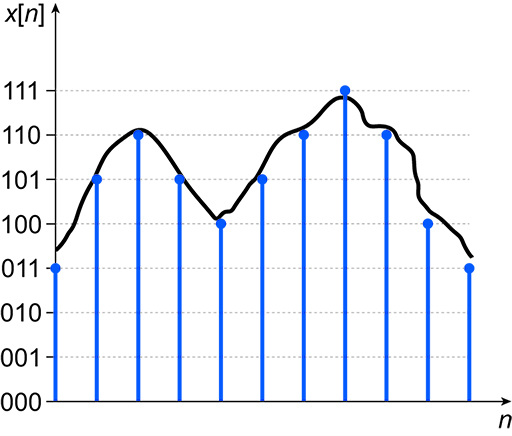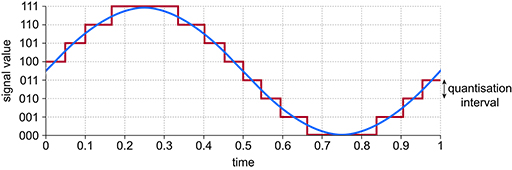Science, Maths & Technology

### Become an OU studentElectronic applications

Start this free course now. Just create an account and sign in. Enrol and complete the course for a free statement of participation or digital badge if available.

# 3.4 Quantisation of a signal

When a continuous-time signal is sampled, the amplitude of each sampled point undergoes quantisation which means that it is forced to have only certain discrete values. The amplitude of each sample is represented by a digital binary code, and the word length of the code will be a fixed number of digital bits. Representing the amplitude of the samples in this way means the value is represented by a binary number, so it is truncated or quantised to its closest binary equivalent.

A 1-bit binary number can represent two levels because it can only take a value of 0 or a value of 1. A 2-bit binary number represents four levels, where each level takes one of the values 00, 01, 10 or 11. Figure 17 shows a discrete-time signal whose values are limited to a 3-bit binary number, which represents eight possible combinations of 0s and 1s – each possible combination is shown on the -axis of the figure. Note that each sampled, digitised signal is a binary representation of the analogue value, so in conversion to these binary representations some rounding of the signal values has occurred: some sampled values are just above and some just below the continuous-time signal.Figure 17 Sampled and quantised signal

Any binary word will always give an even number of quantisation levels. In general, a binary word with bits gives quantisation levels – hence a 3-bit word gives 8 levels, a 4-bit word gives 16 levels, a 5-bit word gives 32 levels, etc.

Figure 18 shows a sine wave along with a set of quantisation levels. Here the 0 or midpoint of the sine wave occurs at a midpoint between the 011 and 100 levels. The gaps between the quantisation levels are called the quantisation intervals. Sometimes a quantisation level is assigned to the 0 or midpoint, which means that on one side of the 0 there is one more level than on the other side.Figure 18 A sine wave and a set of quantisation levels

The larger the quantisation intervals, the more error will be introduced into the sampled signal. The quantisation error for a sample is the difference between the value of the input signal and the resultant quantised signal, with the maximum quantisation error being half the quantisation interval. Quantisation errors can be reduced by increasing the number of levels; however, as the number of levels increases, so does the number of bits needed to represent each sample.

## Activity 7

An electrocardiogram (ECG) signal contains useful information in frequencies up to 400 Hz.

• a.At what rate must the signal be sampled to ensure that no information is lost in sampling?

• b.If a 3-bit quantiser is used to represent the signal, how many bits of data are generated per second if the sampling rate is set to 1000 Hz?

• c.If the signal range from the ECG extends from +7 V to −7 V, where 7 V equates to the highest quantisation level and −7 V to the lowest quantisation level, what is the quantisation interval and what is the maximum quantisation error in the system?

• d.If the quantiser is changed to a 4-bit system, what happens to the maximum quantisation error?